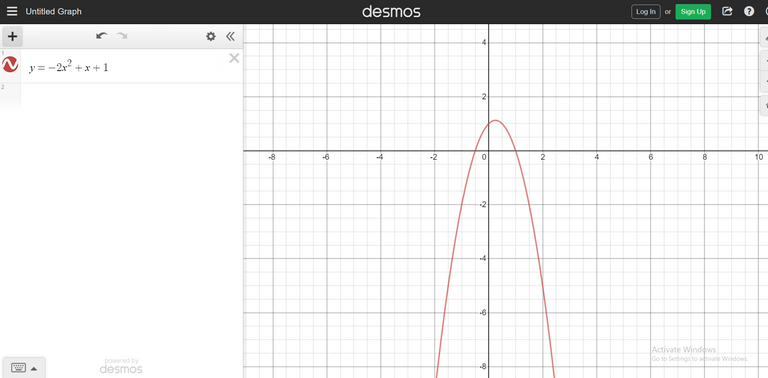# Determining Quadratic Functions From A Table Of Values

in STEMGeeks2 months ago

Hello and welcome. In this mathematics post, I cover the topic of determining quadratic functions given a table of values.

Math text is done with rendered Latex with QuickLatex.com.Pixabay Image Source

## What Is A Quadratic Function?

A quadratic function is a type of mathematical function of the form: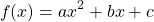where `x` is the independent variable, `f(x)` is the dependent variable that depends on the value of `x`. The coefficients `a`, `b` and `c` are numeric values.

A linear function such as `g(x) = 3x` has `g(x)` being increased by 3 every time `x` is increased by 1. The increase is consistent and does not change every time `x` is increased by 1. With a quadratic function, every time `x` is increased by 1, the amount that `f(x)` increases by does not stay the same.

Let's take a look at some quadratic functions along with their graphs (Screenshots from Desmos). Different quadratic functions will their own shapes in how they open up and down.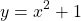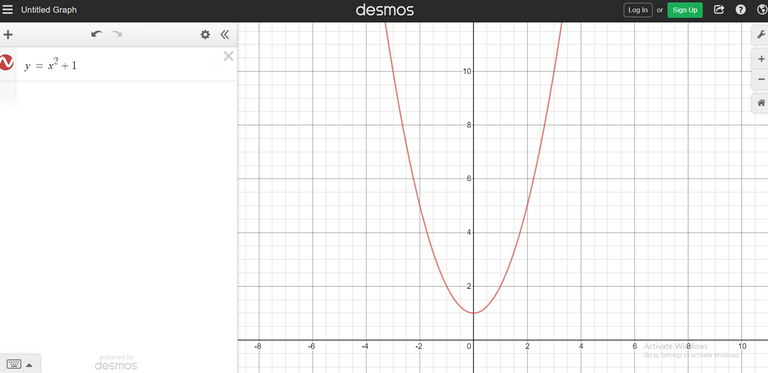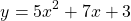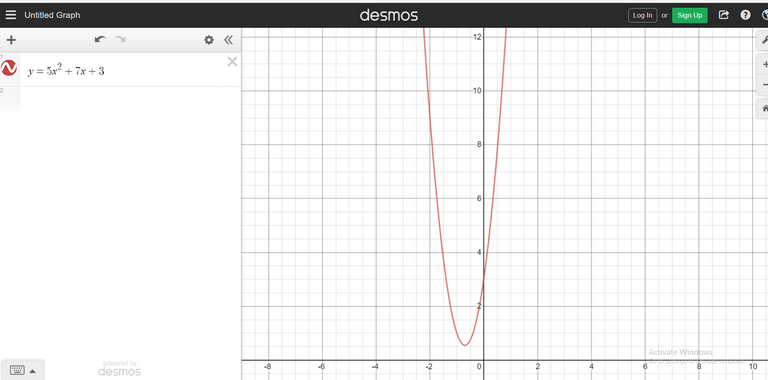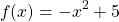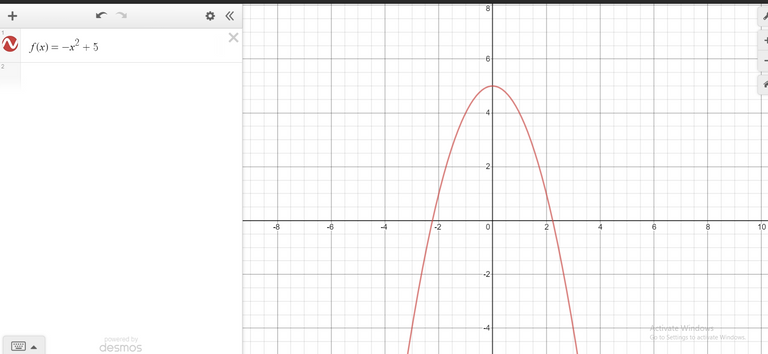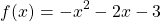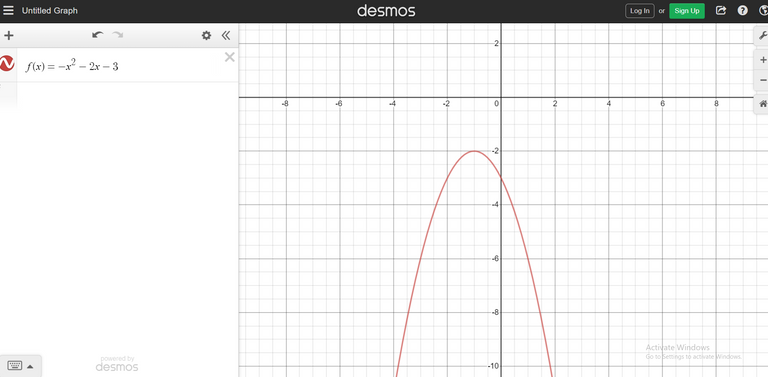## Using Table Of Values To Determine Quadratic Functions

There are times when all you have is just have a table of values. You need to determine if a quadratic function does fit the points from the table of values. If so, what is the quadratic function that does fit the points?

Example One

Here is a table of values for the quadratic function in this example.

xy
-2-1
-1-2
0-1
12
27
314

We need to take a look at the first differences to see if there are the same. First differences takes a y-value minus the previous y-value if it exists.

xyFirst Differences
-2-1N/A
-1-2-1
0-1+1
12+3
27+5
314+7

As the first differences are not the same throughout, the function that fits through these points is not a linear function. Let's take a look at the second differences.

xyFirst DifferencesSecond Differences
-2-1N/AN/A
-1-2-1N/A
0-1+1+2
12+3+2
27+5+2
314+7+2

The second differences are the same which means that the function that fits these points is a quadratic function of the form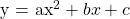. What are the values for the coefficients though?

For the coefficient value of `a`, it is half of the second difference. In this example the value of `a` is half of 2 which is 1.

The value of `c` is the y-intercept. This is the value of `y` when the value of `x` is 0. For this example, the `c` value is -1.

Determining the value of `b` requires algebra along with substituting values from a point from the table in the quadratic function general form. I will use the point (1, 2).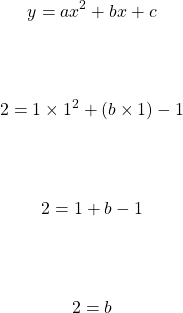The quadratic equation that fits the points in the table of values is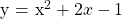.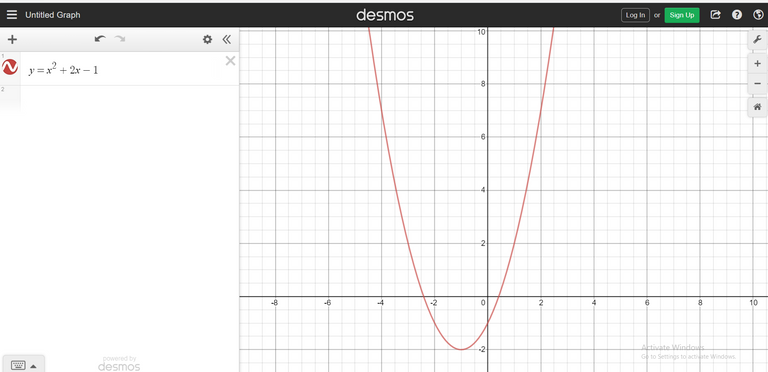Example Two - Not A Quadratic Function

Not every table of values is associated with a quadratic function as shown in this example.

xf(x)
-2-6
-1-3
0-2
1-3
2-7

Taking the first differences and the second differences gives this modified table.

xf(x)First DifferencesSecond Differences
-2-6N/AN/A
-1-3+3N/A
0-2+1-2
1-3-1-2
2-7-4-3

The second differences are not the same. If you find one instance where the second difference is not the same in a table of values, then a quadratic function does not fit through all the points. There is no such quadratic function here.

Example Three

Notice how the last two examples include a y-intercept where there is a y-value when x is equal to 0. This example will show a case of a table of values where there is no y-intercept given.

xg(x)
10
2-5
3-14
4-27
5-44

As `x` increases the amount for `g(x)` decreases. Let's take a look at the first differences and the second differences.

xg(x)First DifferencesSecond Differences
10N/AN/A
2-5-5N/A
3-14-9-4
4-27-13-4
5-44-17-4

The second differences is a constant negative 4. The value for `a` in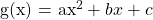is half of -4 which is -2. We now need to determine the values for `b` and `c` for the quadratic function that can fit through these five points. This has to be done with a system of equations.

Select two `(x, y)` points from the table and set up to equations. The first equation uses the x and y value pair from (1, 0) and the second equation uses the x and y pair from the point (2, -5).

First Equation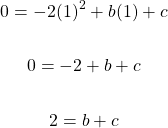Second Equation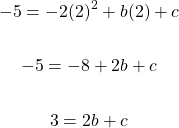To solve this system of equations, you can use the elimination method, a substitution method, or even use matrices from linear algebra. For this example, I will use the substitution method as we can isolate the variable `b` (or `c`) in the first equation.

Start from the first equation: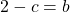Use this value of `b` into the `b` in the second equation. Afterwards, solve for c.

In the second equation: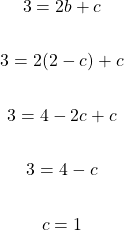The value for `c` is found which can be used to find the value for `b`. I am using equation one to find `b`.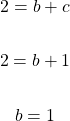A lot has happened in this example. Here is a summary.

xg(x)First DifferencesSecond Differences
10N/AN/A
2-5-5N/A
3-14-9-4
4-27-13-4
5-44-17-4

As the second difference is -4, this makes the value for `a` in the quadratic function half of -4 which is negative 2.

After some algebra, the quadratic function that fits through the (x, y) points above is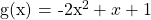.